﻿ 基于EMD的北京与天津PM2.5相关结构探究

# 基于EMD的北京与天津PM2.5相关结构探究Study on Correlation Structures between Beijing and Tianjin PM2.5 Based on EMD

Abstract: In order to explore the correlation between air quality in Beijing and Tianjin, the most representative air quality indexes in Beijing and Tianjin were found to be PM2.5 by calculating the correlation coefficient matrix. Empirical mode decomposition (EMD) was used to decompose the PM2.5 time series in Beijing and Tianjin, and modal reconstruction was carried out based on the period. The results show that PM2.5 in Beijing and PM2.5 in Tianjin are highly correlated in trend terms and strongly correlated in high-frequency parts. In the low-frequency part of PM2.5, Beijing lags behind Tianjin, and the two sequences reach the maximum positive correlation when lagging 1 day.

1. 引言

2. 数据与方法

2.1. 数据来源

2.2. 研究方法

2.2.1. EMD分解

1) 对于给定的时间序列 $x\left(t\right)$ 找到其局部极大值 ${e}_{\mathrm{max}}\left(t\right)$ 和局部极小值 ${e}_{\mathrm{min}}\left(t\right)$ ，构造上包络和下包络，分别用三次样条局部极大值和局部极小值算法；

2) 估计两个包络的平均值 ${M}_{1}\left(t\right)=\frac{{e}_{\mathrm{max}}\left(t\right)+{e}_{\mathrm{min}}\left(t\right)}{2}$

3) 令 ${h}_{1}\left(t\right)=x\left(t\right)-{M}_{1}\left(t\right)$ ，若 ${h}_{1}\left(t\right)$ 满足上述两个条件，则为第一个IMF (固有模态函数)，如果 ${h}_{1}\left(t\right)$ 不满足上述条件，将其作为新的时间序列重复以上步骤直到第k次，即 ${h}_{1k}\left(t\right)$ 为固有模态函数，则：

4) 一直重复前面步骤，直到 $r\left(t\right)$ 为单调函数或者常数，EMD分解结束.这样，原始信号 $X\left(t\right)$ 被分解成 $n-1$ 个固有模态函数和趋势项： $X\left(t\right)={\sum }_{m=1}^{n-1}{C}_{m}\left(t\right)+r\left(t\right)$

2.2.2. 互相关函数

3. 分析与讨论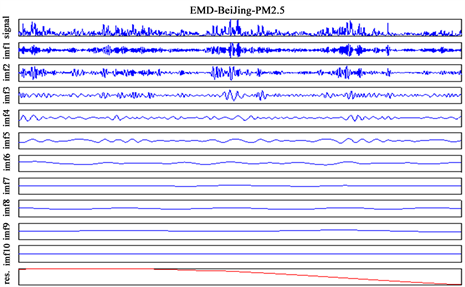(a)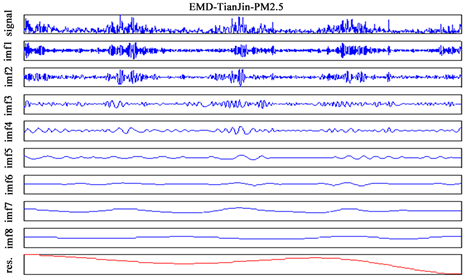(b)

Figure 1. EMD diagram of Beijing PM2.5 and Tianjin PM2.5 time series. (a) EMD results of PM2.5 time series in Beijing; (b) EMD results of PM2.5 time series in TianjinTable 1. IMF cycles of PM2.5 in BeijingTable 2. IMF cycles of PM2.5 in TianjinTable 3. IMF correlation and correlation test between Beijing and Tianjin PM2.5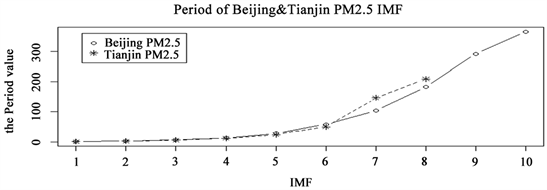Figure 2. Comparison of IMF cycles of PM2.5 in Beijing and Tianjin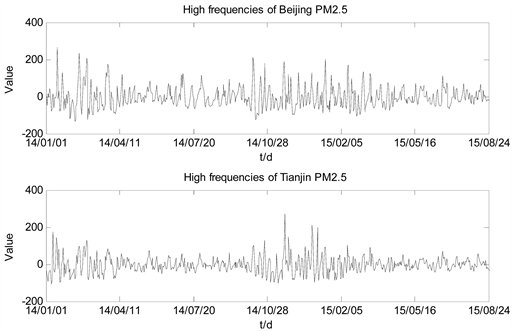Figure 3. Comparison of PM2.5 high-frequency parts between Beijing and Tianjin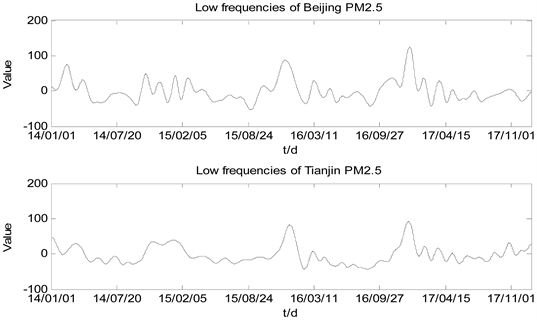Figure 4. Comparison of PM2.5 low-frequency parts between Beijing and TianjinTable 4. Cross correlation results of reconstructed PM2.5 parts in Beijing and Tianjin

4. 结论与讨论

 张纯, 张世秋. 大都市圈的城市形态与空气质量研究综述: 关系识别和分析框架[J]. 城市发展研究, 2014, 21(9): 47-53.

 张森. 协同视角下京津冀城市空气污染影响因素与治理对策研究[D]: [硕士学位论文]. 北京: 首都经济贸易大学, 2017.

 马丽梅, 张晓. 区域大气污染空间效应及产业结构影响[J]. 中国人口, 资源与环境, 2014, 24(7): 157-164.

 马丽梅, 张晓. 中国雾霾污染的空间效应及经济、能源结构影响[J]. 中国工业经济, 2014(4): 19-31.

 David, G.S., Joshua, S.F., Carey, J.J., et al. (2007) Air Quality during the 2008 Beijing Olympic Games. Atmospheric Environment, 41, 480-492.
https://doi.org/10.1016/j.atmosenv.2006.08.046

 王郭臣, 王东启, 陈振楼. 北京冬季严重污染过程的PM2.5污染特征和输送路径及潜在源区[J]. 中国环境科学, 2016, 36(7): 1931-1937.

 董振华, 李芳, 姜愉. EMD以及类EMD方法[J]. 软件导刊, 2014, 13(10): 37-39.

 杨仁树, 付晓强, 杨国梁, 陈骏, 陈玮. EMD和FSWT组合方法在爆破振动信号分析中的应用研究[J]. 振动与冲击, 2017, 36(2): 58-64.

 刘媛媛. 基于整体经验模态分解和随机森林的城市PM2.5预测[D]: [硕士学位论文]. 长春: 长春工业大学, 2017.

 刘玉海, 尹成, 潘树林, 胡永泉. 基于互相关函数法的地面微地震信号检测研究[J]. 石油物探, 2012, 51(6): 633-637.

 董小刚, 周明眉, 秦喜文, 刘媛媛, 张瑜. 基于EMD的PM2.5趋势分析[J]. 长春工业大学学报, 2016, 37(2): 179-181.

 后锐, 伍嘉文, 罗智. 并购浪潮与股市波动:基于EMD的相关性检验与解释[J]. 系统工程, 2013, 31(9): 44-50.

Top